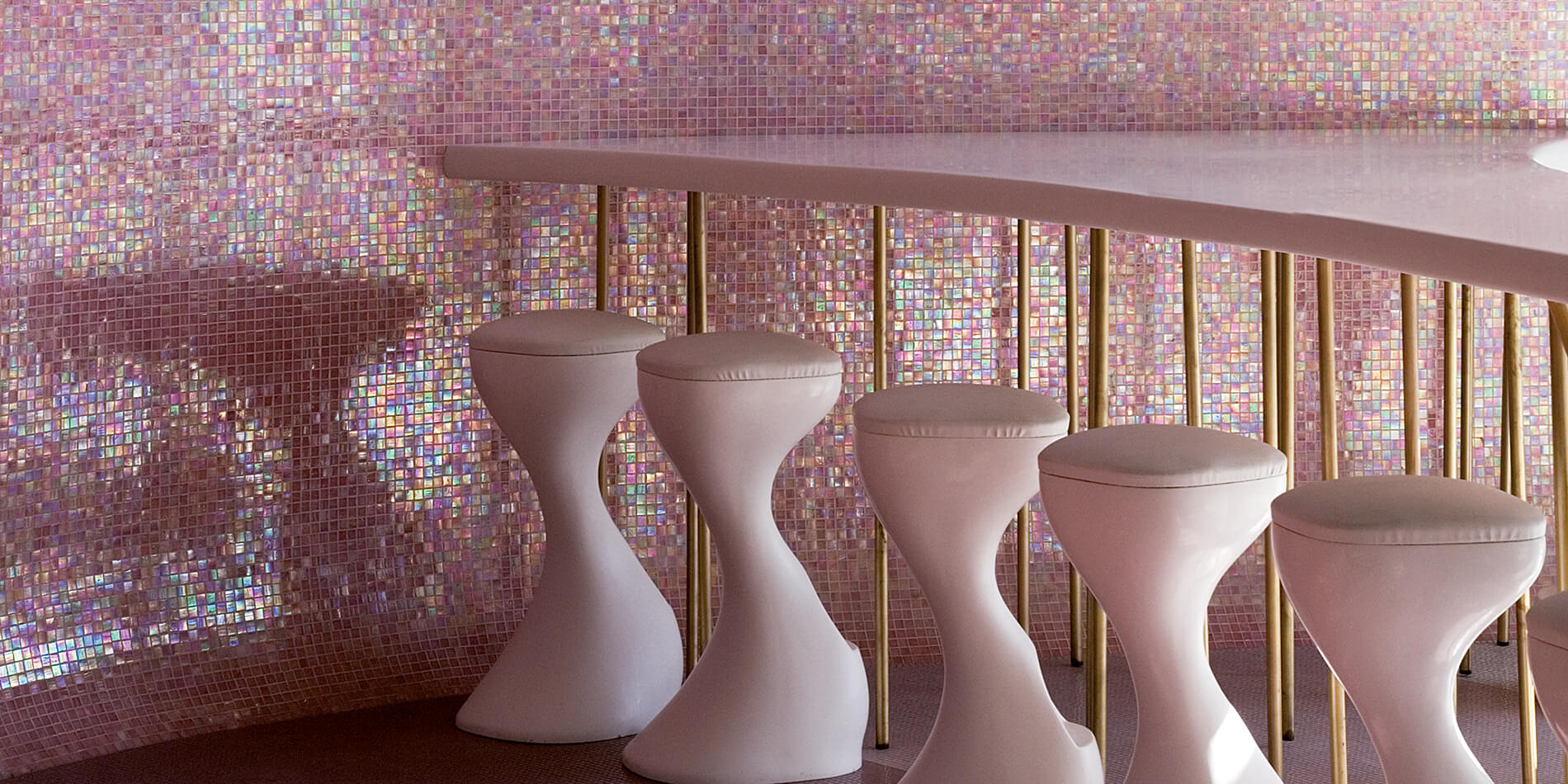### Filter

Content: 1.03 Square meter (€218.38* / 1 Square meter)

€218.38/sqm

Content: 1.03 Square meter (€218.38* / 1 Square meter)

€218.38/sqm

Content: 2.07 Square meter (€91.50* / 1 Square meter)

€91.50/sqm

Content: 2.07 Square meter (€91.50* / 1 Square meter)

€91.50/sqm

Content: 1.03 Square meter (€218.38* / 1 Square meter)

€218.38/sqm

Content: 1.03 Square meter (€218.38* / 1 Square meter)

€218.38/sqm

Content: 2.07 Square meter (€98.82* / 1 Square meter)

€98.82/sqm

Content: 2.07 Square meter (€98.82* / 1 Square meter)

€98.82/sqm

Content: 2.07 Square meter (€96.38* / 1 Square meter)

€96.38/sqm

Content: 2.07 Square meter (€96.38* / 1 Square meter)

€96.38/sqm

Content: 2.07 Square meter (€226.92* / 1 Square meter)

€226.92/sqm

Content: 2.07 Square meter (€226.92* / 1 Square meter)

€226.92/sqm

Content: 1.03 Square meter (€263.52* / 1 Square meter)

€263.52/sqm

Content: 1.03 Square meter (€263.52* / 1 Square meter)

€263.52/sqm

Content: 1.03 Square meter (€263.52* / 1 Square meter)

€263.52/sqm

Content: 1.03 Square meter (€263.52* / 1 Square meter)

€263.52/sqm

Content: 1.03 Square meter (€263.52* / 1 Square meter)

€263.52/sqm

Content: 1.03 Square meter (€263.52* / 1 Square meter)

€263.52/sqm

Content: 0.96 Square meter (€237.90* / 1 Square meter)

€237.90/sqm

Content: 0.96 Square meter (€237.90* / 1 Square meter)

€237.90/sqm

Content: 2.07 Square meter (€226.92* / 1 Square meter)

€226.92/sqm

Content: 2.07 Square meter (€226.92* / 1 Square meter)

€226.92/sqm

Content: 1.29 Square meter (€204.96* / 1 Square meter)

€204.96/sqm

Content: 1.29 Square meter (€204.96* / 1 Square meter)

€204.96/sqm

Content: 2.07 Square meter (€129.32* / 1 Square meter)

€129.32/sqm

Content: 2.07 Square meter (€129.32* / 1 Square meter)

€129.32/sqm

Content: 2.07 Square meter (€129.32* / 1 Square meter)

€129.32/sqm

Content: 2.07 Square meter (€129.32* / 1 Square meter)

€129.32/sqm

Content: 2.07 Square meter (€108.58* / 1 Square meter)

€108.58/sqm

Content: 2.07 Square meter (€108.58* / 1 Square meter)

€108.58/sqm

Content: 2.07 Square meter (€226.92* / 1 Square meter)

€226.92/sqm

Content: 2.07 Square meter (€226.92* / 1 Square meter)

€226.92/sqm

Content: 1.03 Square meter (€263.52* / 1 Square meter)

€263.52/sqm

Content: 1.03 Square meter (€263.52* / 1 Square meter)

€263.52/sqm

Content: 2.07 Square meter (€200.08* / 1 Square meter)

€200.08/sqm

Content: 2.07 Square meter (€200.08* / 1 Square meter)

€200.08/sqm

Content: 2.07 Square meter (€226.92* / 1 Square meter)

€226.92/sqm

Content: 2.07 Square meter (€226.92* / 1 Square meter)

€226.92/sqm

Content: 2.07 Square meter (€226.92* / 1 Square meter)

€226.92/sqm

Content: 2.07 Square meter (€226.92* / 1 Square meter)

€226.92/sqm

Content: 1.5 Square meter (€219.60* / 1 Square meter)

€219.60/sqm

Content: 1.5 Square meter (€219.60* / 1 Square meter)

€219.60/sqm

Content: 1.55 Square meter (€272.06* / 1 Square meter)

€272.06/sqm

Content: 1.55 Square meter (€272.06* / 1 Square meter)

€272.06/sqm

Content: 2.07 Square meter (€267.18* / 1 Square meter)

€267.18/sqm

Content: 2.07 Square meter (€267.18* / 1 Square meter)

€267.18/sqm

Content: 1.29 Square meter (€204.96* / 1 Square meter)

€204.96/sqm

Content: 1.29 Square meter (€204.96* / 1 Square meter)

€204.96/sqm

Content: 1.29 Square meter (€245.22* / 1 Square meter)

€245.22/sqm

Content: 1.29 Square meter (€245.22* / 1 Square meter)

€245.22/sqm

Content: 1.29 Square meter (€204.96* / 1 Square meter)

€204.96/sqm

Content: 1.29 Square meter (€204.96* / 1 Square meter)

€204.96/sqm

Content: 1.29 Square meter (€204.96* / 1 Square meter)

€204.96/sqm

Content: 1.29 Square meter (€204.96* / 1 Square meter)

€204.96/sqm

Content: 1.29 Square meter (€245.22* / 1 Square meter)

€245.22/sqm

Content: 1.29 Square meter (€245.22* / 1 Square meter)

€245.22/sqm

Content: 2.07 Square meter (€226.92* / 1 Square meter)

€226.92/sqm

Content: 2.07 Square meter (€226.92* / 1 Square meter)

€226.92/sqm

Content: 1.03 Square meter (€263.52* / 1 Square meter)

€263.52/sqm

Content: 1.03 Square meter (€263.52* / 1 Square meter)

€263.52/sqm

Content: 2.07 Square meter (€226.92* / 1 Square meter)

€226.92/sqm

Content: 2.07 Square meter (€226.92* / 1 Square meter)

€226.92/sqm

Content: 2.07 Square meter (€226.92* / 1 Square meter)

€226.92/sqm

Content: 2.07 Square meter (€226.92* / 1 Square meter)

€226.92/sqm

Content: 2.07 Square meter (€226.92* / 1 Square meter)

€226.92/sqm

Content: 2.07 Square meter (€226.92* / 1 Square meter)

€226.92/sqm

Content: 2.07 Square meter (€226.92* / 1 Square meter)

€226.92/sqm

Content: 2.07 Square meter (€226.92* / 1 Square meter)

€226.92/sqm

Content: 2.07 Square meter (€129.32* / 1 Square meter)

€129.32/sqm

Content: 2.07 Square meter (€129.32* / 1 Square meter)

€129.32/sqm

Content: 2.07 Square meter (€129.32* / 1 Square meter)

€129.32/sqm

Content: 2.07 Square meter (€129.32* / 1 Square meter)

€129.32/sqm

Content: 2.07 Square meter (€108.58* / 1 Square meter)

€108.58/sqm

Content: 2.07 Square meter (€108.58* / 1 Square meter)

€108.58/sqm

Content: 2.07 Square meter (€108.58* / 1 Square meter)

€108.58/sqm

Content: 2.07 Square meter (€108.58* / 1 Square meter)

€108.58/sqm

Content: 2.07 Square meter (€226.92* / 1 Square meter)

€226.92/sqm

Content: 2.07 Square meter (€226.92* / 1 Square meter)

€226.92/sqm

Content: 1.03 Square meter (€263.52* / 1 Square meter)

€263.52/sqm

Content: 1.03 Square meter (€263.52* / 1 Square meter)

€263.52/sqm

Content: 1.03 Square meter (€263.52* / 1 Square meter)

€263.52/sqm

Content: 1.03 Square meter (€263.52* / 1 Square meter)

€263.52/sqm

Content: 1.03 Square meter (€263.52* / 1 Square meter)

€263.52/sqm

Content: 1.03 Square meter (€263.52* / 1 Square meter)

€263.52/sqm